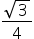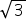Maths-
General
Easy

Question

# Find the area of a piece of cardboard which is in shape of an equilateral triangle of side 12 cm.Hint:

## The correct answer is: 62.35 cm2

### It is given that side of the triangle =12 cmArea of equilateral triangle =(side)2=(12)2  =(144)= 36cm2 (Substituting the value of= 1.73, in the above equation)= 62.35 cm2#### With Turito Foundation.#### Get an Expert Advice From Turito.